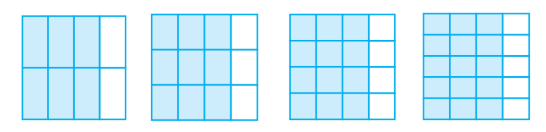## Filters

Sort by :
Q

i need my class 6th admit card for nvs entrance sahil purohit vishakapatnam

What is the last date of Class 12 admission

What is binary number

A binary number is represened by 0 and 1. The binary number system has base 2. It is used in digital electronics. For example 7 can be represented in binary system as 111. That is

Nios board

NIOS is National Institute of Open Schooling and is the board under the Union Government of India.

Calculate the molar mass of the following substances. (c) baking soda

84

Why is glass considered a super cooled liquid?

Glass is an amorphous solid. Just like liquids, amorphous solids also have a tendency to flow, though very slowly. Therefore, these are called pseudo solids or supercooled liquids.

How many stars in milky way?

5

3.20 b) Given the resistances of 1 Ω, 2 Ω, 3 Ω, how will be combine them to get an equivalent resistance of (iii) 6 Ω

Attach them in series

Question 6. Let ∗ be the binary operation on N given by a ∗ b = L.C.M. of a and b. Find

(v) Which elements of N are invertible for the operation ∗?

test

Here is the solution:

Write these in ascending and also in descending order.

(a)

(b)

(c)

option A

Which is the larger fraction?

(i)   or

(ii)   or

(iii)   or

The fractions are shown below using greater than or less than sign                      (i)                           (ii)                         (iii)

You get one-fifth of a bottle of juice and your sister gets one-third of the same size of a bottle of juice. Who gets more?

one third is greater potion than one fifth

Is in its simplest form?

Yes,  is in its simplest form because 49 and 64 has no common divisor.

Write the simplest form of :

(i)

(ii)

(iii)

(iv)

(v)

A

Find five equivalent fractions of each of the following:

(i)

(ii)

(iii)

(iv)

(i)                 (ii)                 (iii)                 (iv)

Identify the fractions in each. Are these fractions equivalent?(i)                                       (ii)                          (iii)                              (iv)  all fractions in it simplest form is   So all fractions are equivalent

Give example of four equivalent fractions.

Four example of  equivalent fractions are :

Are and ;  and   ;  and  and equivalent? Give reason

and ;  and    are not equivalent because    and    but

Fill up using one of these: ‘’, ‘’ or ‘

(a)

(b)

(c)

(d)

(e)

(a)        (b)          (c)       (d)  (e)
Exams
Articles
Questions